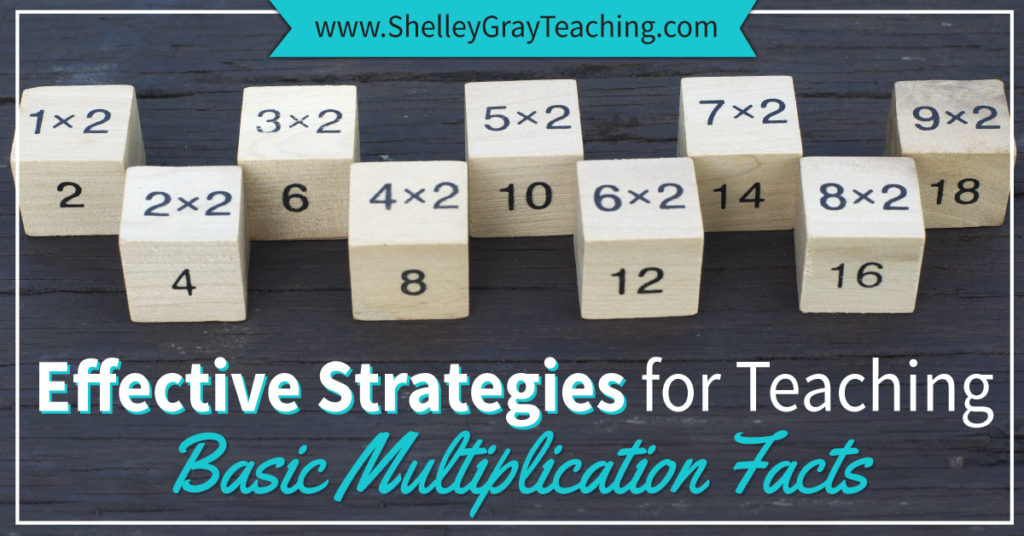# Effective Strategies for Teaching the Basic Multiplication FactsIf you find it hard to teach the basic multiplication facts, you are certainly not alone. There are a few main challenges that we encounter when teaching basic multiplication facts. First – time. How do we find the time to do a really good job of teaching the multiplication facts, while also doing a really good job of teaching everything else in our overwhelming curriculum? We know that basic fact knowledge is essential as students move into the older grades, but it can be difficult to achieve. Secondly, differentiation. All of our students learn at different speeds. We can’t expect them all to learn the multiplication facts at the same time, but how do we ensure that each student is working to his full potential? One last big challenge is the balance between strategies and memorization. Students used to be expected to memorize the facts without any mental math instruction at all. For students who are good at memorization, this works. But there are many students who just can’t memorize all of the facts and remember them. The bigger problem when it comes to memorization is lack of understanding. When we encourage students to simply memorize without making the important connections that they need for conceptual understanding, we are missing a big learning opportunity. It is time for us to take a step back and really ask ourselves what we want our students to be able to do. Do we want them to be able to recite the multiplication facts to 10×10? Or do we want to enable them to solve ANY problem that they encounter – even if it is beyond 10×10. The way you answer this question will ultimately drive your teaching. Fortunately, there are simple shifts that we can make in our teaching to make multiplication easier for our students and accessible for all of them. We can teach in a strategic order that really makes practical sense. We can also teach our students strategies for each set of facts that will make multiplication FUN, and less challenging.
If you are looking for a resource where all of the work is done for you, you may be interested in The Multiplication Station, a self-paced, student-centered math station where students work through the basic multiplication facts and strategies, mastering each one as they go. Strategies are integrated in a strategic manner, ensuring that students build on their understanding progressively. See the Multiplication Station HERE.
Strategies are ESSENTIAL. Think of them like tools in a tool box. When our students see a problem, we want them to be able to choose an effective strategy that will help them solve it. I’ve listed some effective strategies for the facts below. I’ve also listed them in my preferred order of teaching. Simply click on each strategy for a more detailed explanation of each one: The 2’s Facts: Use the doubles addition facts (See a full explanation HERE) The 10’s Facts: Shift the digits one place to the left (See a full explanation HERE) The 5’s Facts: Use the related 10’s fact and divide in half (See a full explanation HERE) The 1’s Facts: Anything times 1 is itself. The 0’s Facts: Anything times 0 is 0. Square Facts: Learn the squares like 3×3, 4×4, 5×5 by using arrays where we can “see” the square that is created. These facts will be important for deriving facts later on. The 4’s Facts: The double of the double (See a full explanation HERE) The 3’s Facts: The double plus one more group (See a full explanation HERE) The 9’s Facts: The related 10’s fact subtract one group (See a full explanation HERE) The 6’s Facts: Use a known fact. (See a full explanation HERE) The 7’s Facts: Use a known fact. (See a full explanation HERE) The 8’s Facts: Use a known fact.(See a full explanation HERE)     Let’s talk a little bit about strategies vs. tricks. One thing to remember is that a strategy will enable students to solve ANY problem, while a trick is limited. Take for example, the “just add a 0” trick for multiplying by 10. Is this effective for multiplying whole numbers by 10? Yes, absolutely it is. But there is a problem. Once students get into multiplying decimals, they will have to un-learn this trick. To solve 13.4 can you just add a 0 and make it 13.40? No. In this case it is more effective to teach students to shift the digits one place to the left, encouraging a place value understanding of multiplying by 10. The next time that you are teaching a strategy, be sure to ask yourself, “Is this a strategy or a trick?”   NEXT STEPS: Read more about an effective order for teaching the multiplication facts HERE. This order will motivate your students and enable them to see huge progress early on in their learning.